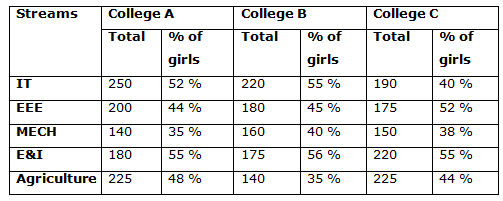# IBPS Clerk Prelims 2018 – Quantitative Aptitude Questions Day-19

Dear Readers, Bank Exam Race for the Year 2018 is already started, To enrich your preparation here we have providing new series of Practice Questions on Quantitative Aptitude – Section. Candidates those who are preparing for IBPS Clerk Prelims 2018 Exams can practice these questions daily and make your preparation effective.

[WpProQuiz 4178]

Directions (Q. 1 – 5) what value should come in place of question mark (?) in the following questions?

1) (4/25) × 38850 + 32% of 750 =? + 2568

a) 3276

b) 3592

c) 3888

d) 4254

e) None of these

2) (4 5/7) ÷ (2 3/4) × (147/152) × 228 =?

a) 378

b) 412

c) 324

d) 456

e) None of these

3) (3150 ÷ 18) + (1705 ÷ 11) + (2555 ÷ 7) =?

a) 734

b) 812

c) 956

d) 695

e) None of these

4) √33856 ÷ 4 + 140608 = 133 + 14 % of 750 – ?

a) 2562

b) 2204

c) 2846

d) 3128

e) None of these

5) ? – 162 ÷ 255 × 3060 + 93 = 18 % of 6600

a) 4857

b) 4179

c) 3531

d) 3323

e) None of these

Directions (Q. 6 – 10) Study the following information carefully and answer the given questions.

The following table shows the total number of students studying in various departments in various engineering colleges in the year 2017 and the percentage of girls among them.6) Find the difference between the total number of boys in EEE and E&I department in college A to that of total number of girls in IT and Agriculture department in college C?

a) 18

b) 29

c) 35

d) 44

e) None of these

7) Find the ratio between the total number of students in MECH department to that of E & I department in all the given colleges together?

a) 15: 32

b) 27: 49

c) 18: 23

d) 53: 69

e) None of these

8) Total number of girls in IT department in college B is approximately what percentage of total number of boys in MECH department in college C?

a) 115 %

b) 130 %

c) 90 %

d) 145 %

e) 75 %

9) Find the average number of girls in IT, MECH and Agriculture department in college B?

a) 124

b) 180

c) 152

d) 78

e) None of these

10) Total students in college A is approximately what percentage more/less than the total students in college C in all the given departments together?

a) 15 % less

b) 4 % more

c) 15 % more

d) 22 % less

e) 4 % less

(4/25) × 38850 + (32/100)*750 = x + 2568

6216 + 240 – 2568 = x

X = 3888

(33/7)*(4/11)*(147/152)*228 = x

X = 378

(3150 ÷ 18) + (1705 ÷ 11) + (2555 ÷ 7) = x

X = 175 + 155 + 365 = 695

√33856 ÷ 4 + ∛140608 = 133 + 14 % of 750 – x

(184/4) + 52 = 2197 + (14/100)*750 – x

x = 2197 + 105 – 46 – 52

x = 2204

x – 162 ÷ 255 × 3060 + 93 = 18 % of 6600

x – (16*16*3060)/255 + 729 = (18/100)*6600

x = 1188 + 3072 – 729 = 3531

Direction (6-10) :

The total number of boys in EEE and E&I department in college A

= > 200*(56/100) + 180*(45/100)

= > 112 + 81 = 193

The total number of girls in IT and Agriculture department in college C

= > 190*(40/100) + 225*(44/100)

= > 76 + 99 = 175

Required difference = 193 – 175 = 18

The total number of students MECH department in all the given colleges together

= > 140 + 160 + 150 = 450

The total number of students E&I department in all the given colleges together

= > 180 + 175 + 220 = 575

Required ratio = 450: 575 = 18: 23

Total number of girls in IT department in college B

= > 220*(55/100) = 121

Total number of boys in MECH department in college C

= > 150*(62/100) = 93

Required % = (121/93)*100 = 130 %

The total number of girls in IT, MECH and Agriculture department in college B

= > 220*(55/100) + 160*(40/100) + 140*(35/100)

= > 121 + 64 + 49 = 234

Required average = 234/3 = 78

Total students in college A in all the given departments together

= > 250 + 200 + 140 + 180 + 225 = 995

Total students in college C in all the given departments together

= > 190 + 175 + 150 + 220 + 225 = 960

Required % = [(995 – 960)/960]*100 = 4 % more

Daily Practice Test Schedule | Good Luck

 Topic Daily Publishing Time Daily News Papers & Editorials 8.00 AM Current Affairs Quiz 9.00 AM Current Affairs Quiz (Hindi) 9.30 AM IBPS Clerk Prelims – Reasoning 10.00 AM IBPS Clerk Prelims – Reasoning (Hindi) 10.30 AM IBPS Clerk Prelims – Quantitative Aptitude 11.00 AM IBPS Clerk Prelims – Quantitative Aptitude (Hindi) 11.30 AM Vocabulary (Based on The Hindu) 12.00 PM IBPS PO Prelims – English Language 1.00 PM SSC Practice Questions (Reasoning/Quantitative aptitude) 2.00 PM IBPS PO/Clerk – GK Questions 3.00 PM SSC Practice Questions (English/General Knowledge) 4.00 PM Daily Current Affairs Updates 5.00 PM IBPS PO Mains – Reasoning 6.00 PM IBPS PO Mains – Quantitative Aptitude 7.00 PM IBPS PO Mains – English Language 8.00 PM